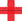top of page

## Video ResourcesIn this video, Luke or Lindsey takes students through a full Stats Medic EFFL lesson.

# Sample Proportions

##### Learning Targets
• Calculate the mean and standard deviation of the sampling distribution of a sample proportion and interpret the standard deviation.

• Determine if the sampling distribution of a sample proportion is approximately Normal.

• If appropriate, use a Normal distribution to calculate probabilities involving a sample proportion.

bottom of page